Function Repository Resource:

# IsContinuousFunction

Determine whether a function is continuous

Contributed by: Wolfram|Alpha Math Team
 ResourceFunction["IsContinuousFunction"][expr,x,pt] assesses whether expr is continuous, viewed as a real-valued function of x, at the point x=pt. ResourceFunction["IsContinuousFunction"][expr,x] assesses whether expr is continuous over the full set of real numbers. ResourceFunction["IsContinuousFunction"][{expr,cond},x] assesses whether expr is continuous, subject to condition cond.

## Details and Options

A function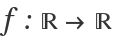is continuous at a point c if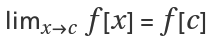. The function f is continuous on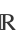if it is continuous at every point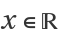.

## Examples

### Basic Examples (2)

Test if a function is continuous over the Reals:

 In:=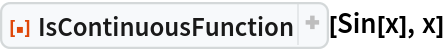Out=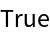Test if a function is continuous at a point:

 In:=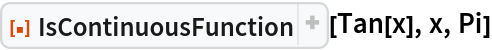Out=In:=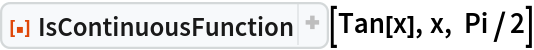Out=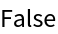### Scope (2)

One can explicitly specify a domain over which to test continuity:

 In:=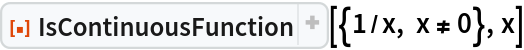Out=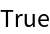Omitting the condition on x produces a different result:

 In:=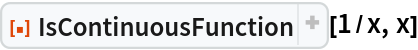Out=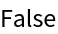### Properties and Relations (1)

IsContinuousFunction uses the resource function FunctionDiscontinuities to determine the points of discontinuity of an expression:

 In:=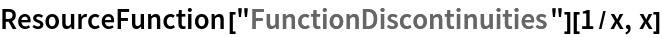Out=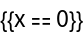## Publisher

Wolfram|Alpha Math Team

## Version History

• 3.0.0 – 23 March 2023
• 2.0.0 – 23 July 2020
• 1.0.0 – 23 July 2020

## Author Notes

To view underlying paclet code, evaluate the following:

 In:=